## Material for unsaturated flow in lattice models - LatticeTransMat

Lattice transport elements have to be used with this material model. A positive sign is assumed for liquid tension, unlike the convention of soil mechanics which assumes compression positive.

These transport elements are idealised as one-dimensional conductive pipes. The gradient of hydraulic head, which governs flow rate along each transport element, is determined from the capillary pressures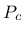at the two nodes.

The mass balance equation describes the change in moisture inside a porous element as a consequence of liquid flow and solid-liquid retention. It leads to the following partial differential equation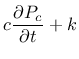div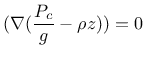(267)

whereis the capillary pressure,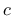is the mass capacity function(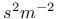),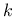is the Darcy hydraulic conductivity (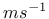),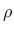is the fluid mass density,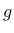is the acceleration of gravity,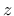is the capillary height and t is the time.

The hydraulic conductivityconsists of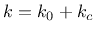(268)

where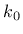is the hydraulic conductivity of the intact material and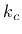is the additional conductivity due to cracking.

Darcy hydraulic conductivityis defined as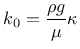(269)

where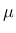is the dynamic viscosity (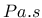),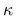is the permeability also called intrinsic conductivity(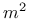), and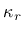is the relative conductivity.is a function of the effective degree of saturation.

The cracking part is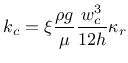(270)

where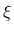is a tortuosity factor taking into account the roughness of the crack surface,is the equivalent crack opening of the dual mechanical lattice and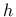is the length of the dual mechanical element.

Subsections
Borek Patzak
2019-03-19